I have been asked to create a classifier to identify the speaker of recordings of individual phrases spoken by either Di or Souhaib. Each sound sample consists of 1,000 amplitude values, which have been downsampled from the original recordings. For my final classifier, I have researched and exploited some of the properties of frequency spectra of sound waves, as well as other techniques in audio analysis.

Before we embark on this project, though:

``set.seed(7) #For luck!``

# Data Exploration

First, let’s plot some of our training data.

``marrangeGrob(basic_plots, nrow=2, ncol=1, top=NULL)``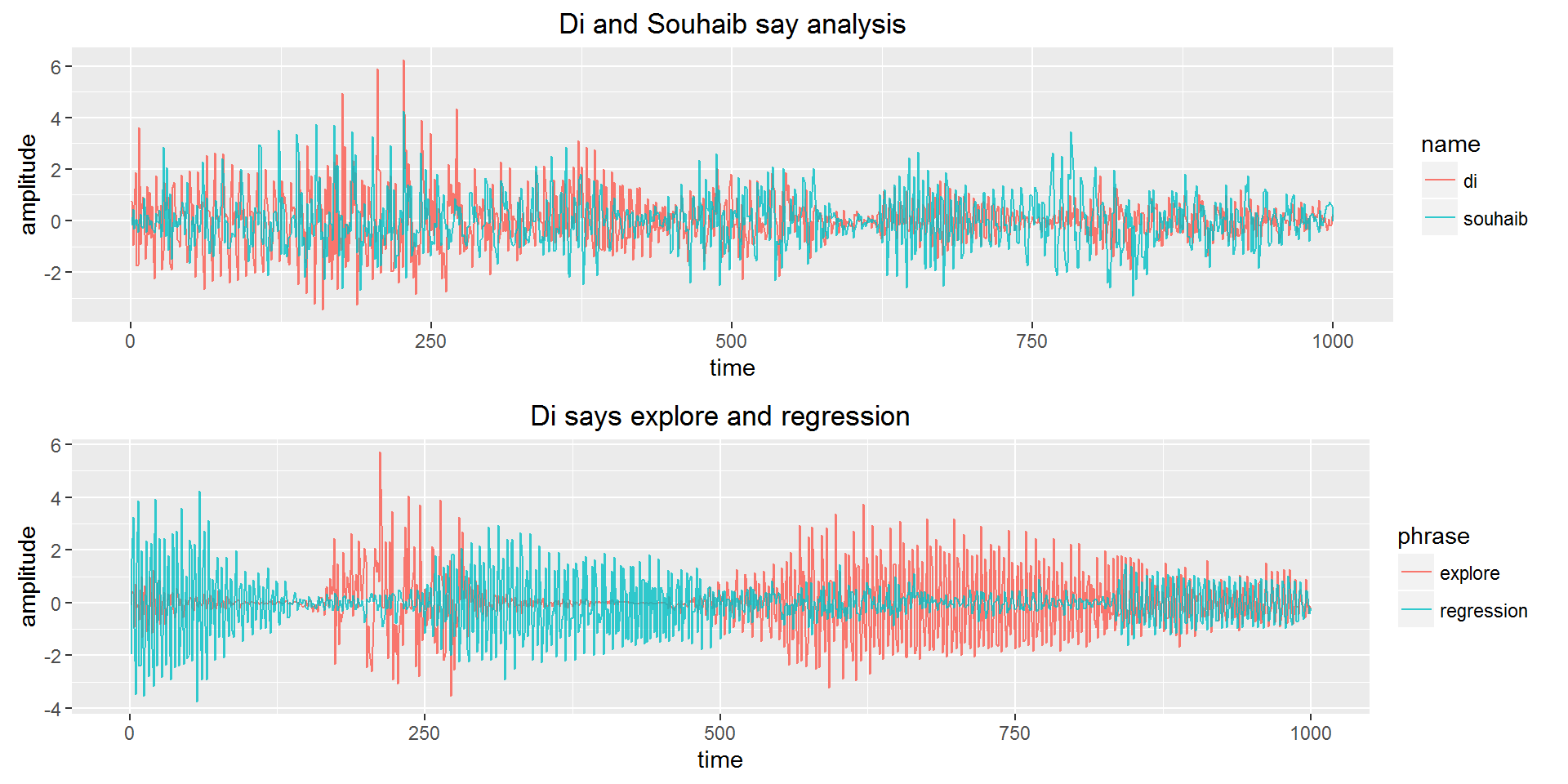``plot_training_words(tr_long)``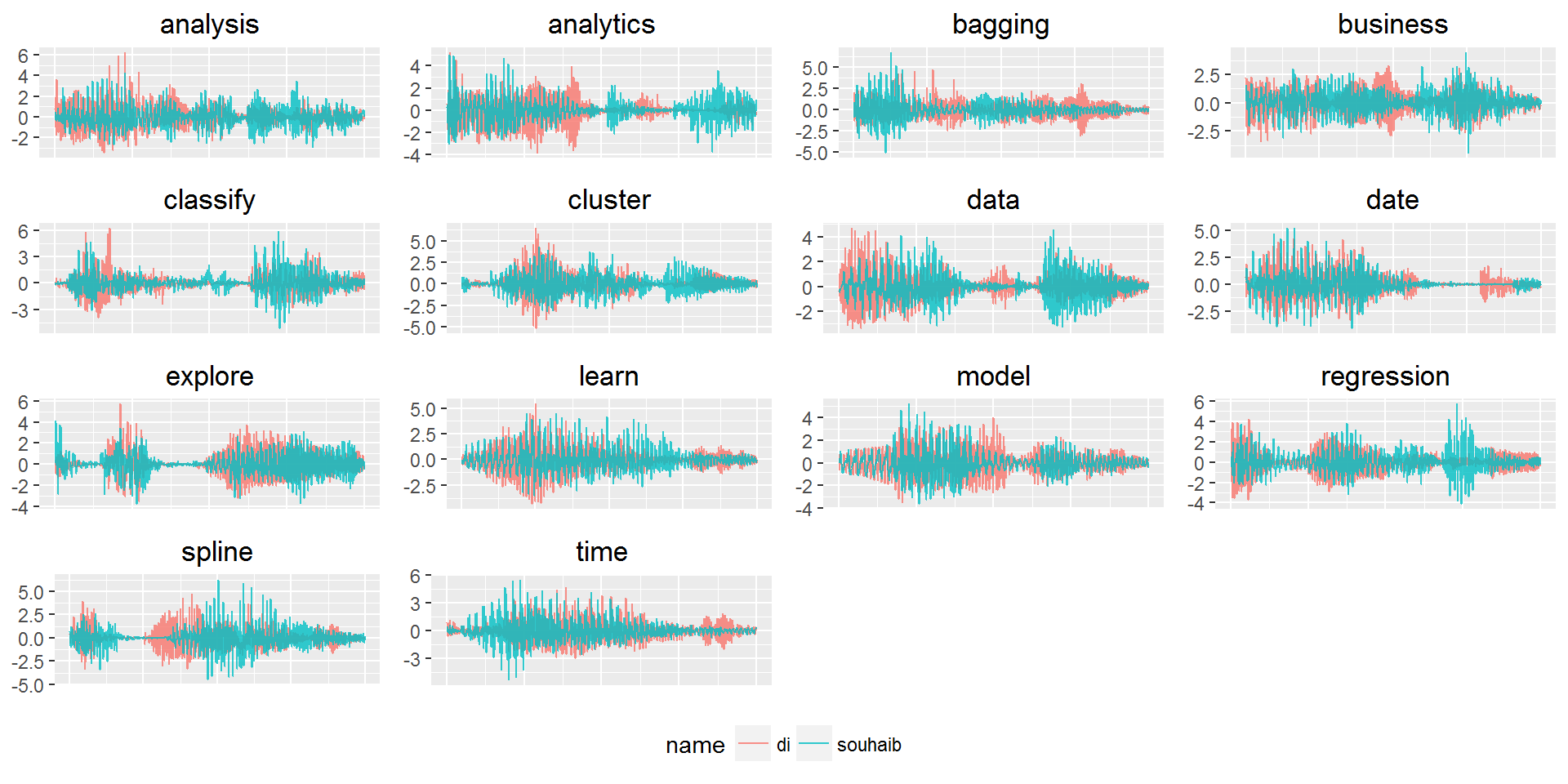A problem is immediately evident. It appears the waveform of the same word spoken by Di and Souhaib have a relatively high covariance, compared with two different words spoken by the same speaker. This pattern is evident in the full training data, plotted as overlaid waveforms:

``````ggplot(tr_long, aes(time, value, colour=name, alpha=name)) +
geom_line() +
scale_alpha_discrete(range=c(1, 0.7)) +
labs(title="All waveforms overlaid", y="amplitude")``````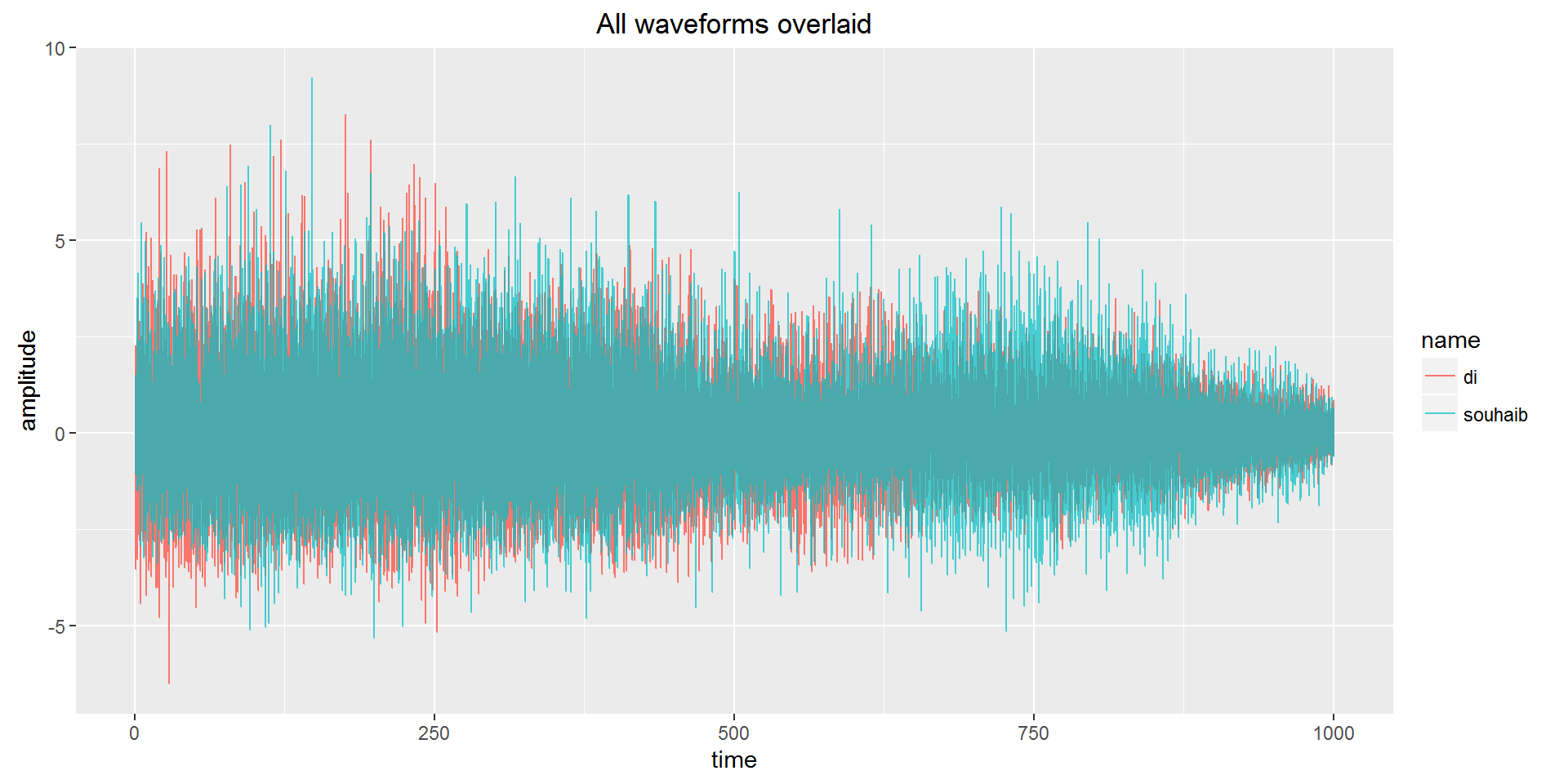We will therefore need to try to extract features of the data that have low variance between different words, but high variance between different speakers.

Before transforming our data however, let’s see if there are any sections of the raw waveforms that have any predictive power. We will define a function, `separation_plot()`, that takes a two column data frame, fits a linear Support Vector Machine, and plots the resulting classifier. This will allow us to rapidly investigate potential features.

We will define `tr` as the waveforms of the training data, and `ts` as the waveforms of the test data.

## Classification using the raw waveform

First up: by eyeballing the above waveform plot, the following separation was found on the raw waveforms. Note that we are only considering the absolute values of the waveforms, as a negative value on a waveform represents the same amplitude as a positive value.

When we create each linear SVM classifier, we will also keep a running count of wrong predictions in the training set, `wrong_tally`.

``````amplitude_separation <- data.frame(apply(tr, 1, function(x){mean(subset(abs(x[600:825]), abs(x[600:825]) > .1))}),
apply(tr, 1, function(x){mean(x[-(600:825)])}))
separation_plot(amplitude_separation,
"Mean of amplitudes > 0.1 at 599 < time < 826",
"Mean of remaining amplitudes")``````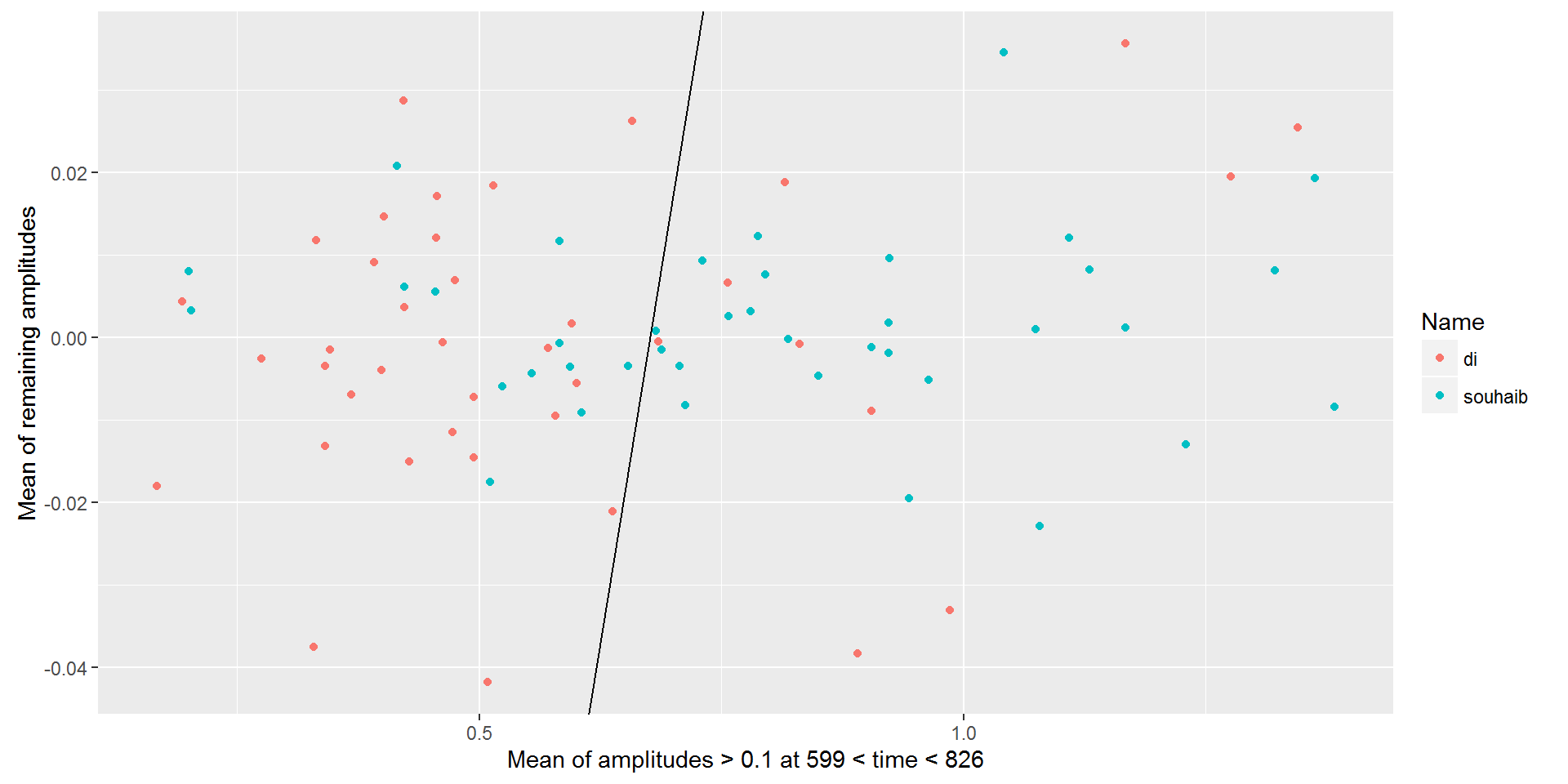``````svm_model <- svm(name~., data.frame(amplitude_separation, name=tr_answers), kernel="linear")
wrong_tally <- as.numeric(predict(svm_model, amplitude_separation) != tr_answers)``````

Interestingly it appears that we can achieve a fairly good separation using the mean of some of the amplitudes of the waveforms alone. As expected however, when these are excluded, the remaining amplitudes plotted on the y-axis are next to useless for classification.

We can incorporate more of this information in the simple 2-variable plot using principal components.

``````pca <- prcomp(abs(tr))
pca_separation <- data.frame(
colSums(abs(apply(tr, 1, function(x){as.numeric(pca\$rotation[,1]*abs(x))}))),
colSums(abs(apply(tr, 1, function(x){as.numeric(pca\$rotation[,2]*abs(x))})))
)
separation_plot(pca_separation, "PC1", "PC2")``````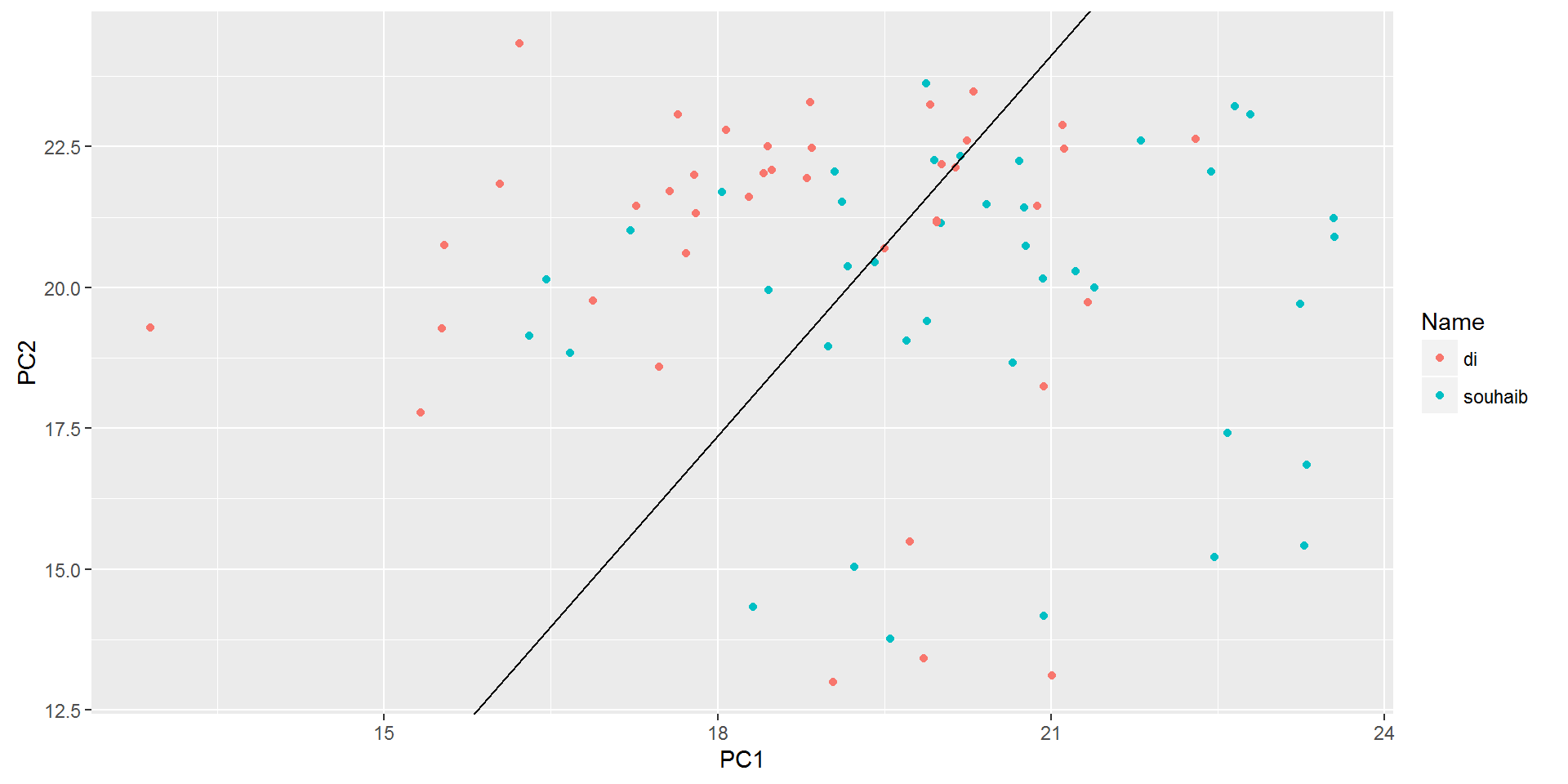``wrong_tally <- update_tally(pca_separation, wrong_tally)``

Once again, we see that the absolute value of the waveforms give a fairly good split of the data, so we should include this in our model.

# Transformation and Feature Extraction

## The Fourier Transform

We can think of our waveforms as essentially continuous time series. An important mathematical transformation for continuous functions over time is the Fourier Transform, which converts a signal from a function of time to a function of frequency. Therefore, by taking the Fourier Transform of each signal, we will get a representation of the relative power of a series of sine waves in the additive signal.

The Fourier Transform returns a real and a complex element. The complex element represents the phase shift of each component frequency, which is of little use in our analysis. We will only consider the absolute magnitude of the Fourier Transform, which represents the magnitude of each component sine wave.

This is likely to be a very powerful technique for feature extraction, as Souhaib has a deeper voice than Di, so the distribution of the Fourier Transform of each of Souhaib’s words is likely to be more positively skewed than Di’s.

One thing to note: because of the way the test data was encoded, the frequency of each sample is variable. An important implication of this is that our Fourier Transforms can only be considered rough estimates of the “true” frequency distribution.

To illustrate this problem, when listening to the recordings, we notice:

``````listen(as.numeric(tr[1,]), f=1400)  # Di: analysis. The pitch sounds approximately correct at f = 1400.
listen(as.numeric(tr[75,]), f=1650)  # Di: time. This word is shorter, so requires a higher frequency rate = 1650 to sound right.``````
1. To achieve a correct pitch for Di saying analysis, we set the frequency at around 1400.
2. To achieve a correct pitch for Di saying time, we set the frequency at around 1650.

We will later consider ways to remove this variation between samples.

As before, let’s look at the plots of the Fourier Transform on a sample of each of our words:

``````fft_wide <- cbind(tr_wide[,1:4], t(apply(tr, 1, function(x){abs(fft(x))}))[,1:500])
fft_long <- melt(fft_wide, id.var=c("id", "name", "phrase", "rep"), variable.name="time")
fft_long\$time <- rep(1:500, each=80)
fft_long\$value <- sqrt(fft_long\$value)

plot_training_words(fft_long)``````